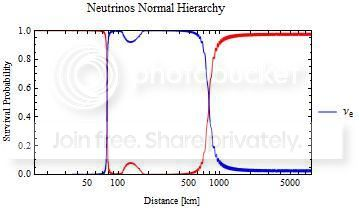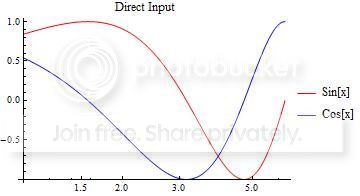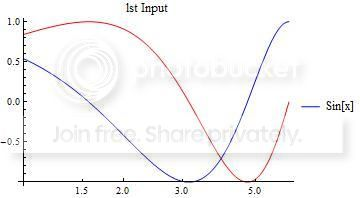# Plot legends not displaying correctly

I'm having an issue with a notebook I'm running. I'm generating a bunch of plots, but the legends aren't showing up correctly. I generate a bunch of plots every time I run the program so I wrote a Module to make all the plots with one line rather than type it out over and over. The Module works right except for the plot legends.

Plots[o_, f_] := Module[{style, legends, alegends, a, b, c, d, e},
style = If[n == 2, {Red, Blue}, {Red, Blue, Green}];
legends = If[n == 3, {"ve","vm","vt"},If[n == 2,
If[m == 2, {"ve","vm"}, {"ve","vt"}], If[n == 4, {"ve", "vm", "vt", "vs"}, "error"]]];
alegends = If[n == 3, {"vebar","vmbar","vtbar"},
If[n == 2, If[m == 2, {"vebar","vmbar"}, {"vebar","vtbar"}],
If[n == 4, {"vebar", "vmbar", "vtbar", "vsbar"}, "error"]]];
a = LogLinearPlot[NN, {x, o, f}, PlotStyle -> style, PlotRange -> {{0, f}, {0, 1}},
Frame -> True, FrameLabel -> {"Distance [km]", "Survival Probability"},
PlotLegends -> Placed[legends, {1, 0.5}], PlotLabel -> "Neutrinos Normal Hierarchy"];
b = LogLinearPlot[NA, {x, o, f}, PlotStyle -> style, PlotRange -> {{0, f}, {0, 1}},
Frame -> True, FrameLabel -> {"Distance [km]", "Survival Probability"},
PlotLegends -> Placed[alegends, {1, 0.5}], PlotLabel -> "Anti-Neutrinos Normal Hierarchy"];
c = LogLinearPlot[IN, {x, o, f}, PlotStyle -> style, PlotRange -> {{0, f}, {0, 1}},
Frame -> True, FrameLabel -> {"Distance [km]", "Survival Probability"},
PlotLegends -> Placed[legends, {1, 0.5}], PlotLabel -> "Neutrinos Inverted Hierarchy"];
d = LogLinearPlot[IA, {x, o, f}, PlotStyle -> style, PlotRange -> {{0, f}, {0, 1}},
Frame -> True, FrameLabel -> {"Distance [km]", "Survival Probability"},
PlotLegends -> Placed[alegends, {1, 0.5}], PlotLabel -> "Anti-Neutrinos Inverted Hierarchy"];
CellPrint@ExpressionCell[#, "Output"] & /@ {a, b, c, d, e};
]


This returns a plots that look like the following:As you can see I get the plot, the styling, the frame, the labels, but the legend only has "ve" and it doesn't correspond to the correct color -- the key is blue with "ve" but the plot has "ve" red and "vt" blue.

Any help with this would be greatly appreciated.

EDIT: n and m are inputs. n determines the number of flavors of particles I'm considering (in the listed case n = 2) and m determines which two I'm working with (in this case m = 3 so I'm looking at the 1st and 3rd flavor).

EDIT 2: Thanks to the answer by Verbeia and playing around a little I have realized that the problem isn't in the Plot Legends exactly. It's in the primary argument. Since the functions I'm plotting are list of size n (a parameter set within the program) and are output from another function I pass them to the LogLinearPlot function as the name of the list. However I've found that if you do that the legends doesn't behave correctly.

Consider the following:

lst = {Sin[x],Cos[x]};
LogLinearPlot[{Sin[x],Cos[x]},{x,1,2$\pi$},PlotStyle->{Red,Blue},PlotLegends->Placed[{"Sin[x]","Cos[x]"},{1,0.5}]
LogLinearPlot[lst,{x,1,2$\pi$},PlotStyle->{Red,Blue},PlotLegends->Placed[{"Sin[x]","Cos[x]"},{1,0.5}]


This produces the following two images:The problem in my case is that the list of functions NN are the outputs of other functions in the program and since my code is designed to handle a general case of n = {2,3,4} I can't just list out my functions.

• Welcome to Mathematica.SE! I hope you will become a regular contributor. To get started, 1) take the introductory tour now, 2) when you see good questions and answers, vote them up by clicking the gray triangles, because the credibility of the system is based on the reputation gained by users sharing their knowledge, 3) remember to accept the answer, if any, that solves your problem, by clicking the checkmark sign, and 4) give help too, by answering questions in your areas of expertise. – bbgodfrey Oct 8 '15 at 2:26
• Please provide some sample input for Plots, so that the reader can run your code. It is difficult to solve a problem without being able to reproduce it. Also, what is the value of n? – bbgodfrey Oct 8 '15 at 2:31

As bbgodfrey pointed out, it is a bit difficult to identify the issue without knowing what n is and what m is, but I suspect that n is an attempt to capture the number of functions being plotted. If so, you probably should not have this as a global constant, but instead either capture it based on the data you are passing to the LogLinearPlot functions or pass it as another parameter to your Plots function (i.e. change the definition of Plots). The same applies for m.

I think you would get a better outcome if you defined your legends and alegends expressions as a function of n, outside the Plots definition. You could also use Which or Switch to make the logic easier to read.

Something like this (assuming you have separately defined m=2):

legends[n_Integer?Positive]:= Switch[n , 3, {"ve","vm","vt"},
2, If[m == 2, {"ve","vm"}, {"ve","vt"}], 4, {"ve", "vm", "vt", "vs"}, _,"error"]


You would then use the PlotLegends option like this:

PlotLegends -> Placed[legends, {1, 0.5}]


Here is an example, obviously not your actual data:

 LogLinearPlot[{Tanh[x], x^(3/2)}, {x, 1, 100},
PlotStyle -> {Red, Blue}, PlotRange -> {{0, 100}, {0, 100}}, Frame -> True,
FrameLabel -> {"Distance [km]", "Survival Probability"},
PlotLegends -> Placed[legends, {1, 0.5}],
PlotLabel -> "Anti-Neutrinos Normal Hierarchy"]As you can see, the legend works fine in this case, provided m has already been defined.

As an aside, there is no need to separately identify different cases for your styles as long as the first line is always red, the next blue or whatever. The plot above is unchanged if I write PlotStyle -> {Red, Blue, Green, Purple} instead of PlotStyle -> {Red, Blue}.

# Edit

The edit to your question demonstrates how important it is to provide complete information.

I have established that this is a version-specific issue, and it's specific to LogLinearPlot. I can reproduce your legending issue on version 9. On version 10.1, it all works fine.

I therefore think this might be a bug. I suggest you contact Wolfram Support for confirmation, and then add the tag to this question if they confirm it. The fix is to upgrade to the latest version of Mathematica.

Version 9

myfun = {Sin[x], Cos[x]};
Plot[myfun, {x, 0.1, 2 Pi},
PlotLegends -> Placed[{"This", "That"}, {1, 0.5}]]LogLinearPlot[myfun, {x, 0.1, 2 Pi},
PlotLegends -> Placed[{"This", "That"}, {1, 0.5}]]Version 10.1

myfun = {Sin[x], Cos[x]};
Plot[myfun, {x, 0, 2 Pi}, PlotLegends -> {"This", "That"}]LogLinearPlot[myfun, {x, 0.1, 2 Pi},
PlotLegends -> Placed[{"This", "That"}, {1, 0.5}]]